# Vertex Definition

Vertex Definition

Vertex is the point where two or more lines or edges meet to form an angle. In this lesson, you will learn about vertex examples, the vertex definition, and the vertex angle.

Let's have a look at the two rays: Ray 1 and Ray 2, they originate from the vertex O.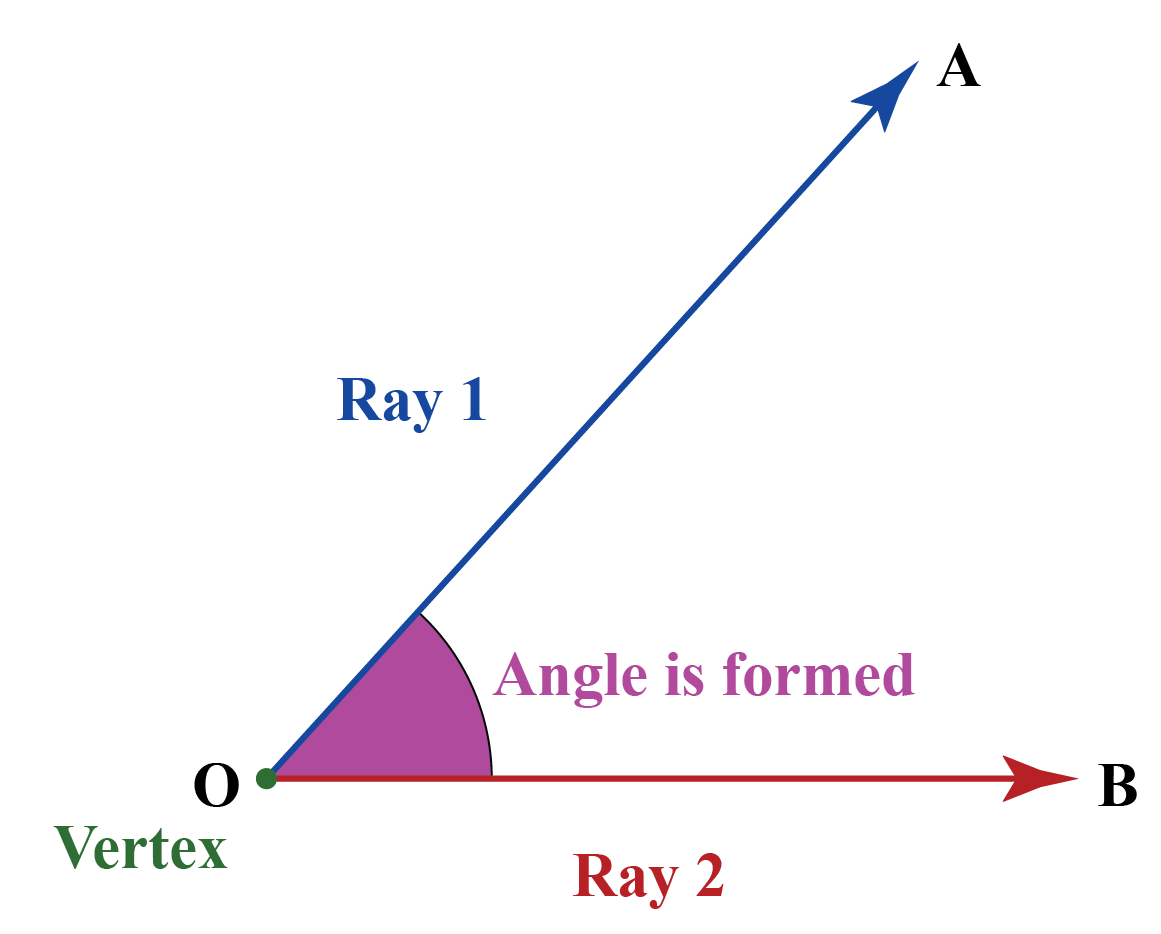As we go forward, you can check out the interactive examples to know more about the lesson and try your hand at solving a few interesting practice questions at the end of the page.

Learn all you need to know about vertex in this short lesson!

## Lesson Plan

 1 What Is a Vertex? 2 Solved Examples on Vertex 3 Interactive Questions on Vertex

## What Is a Vertex?

A vertex in math is a point where two lines or rays meet.

An angle is formed at the vertex.

A vertex is denoted by uppercase letters like A, O, P, etc.

The plural of vertex is "vertices." In solid geometry, i.e., three-dimensional geometry, shapes such as cubes, cuboids form several vertices. Let us look at them in detail in the next section.

Look at the triangle DEF.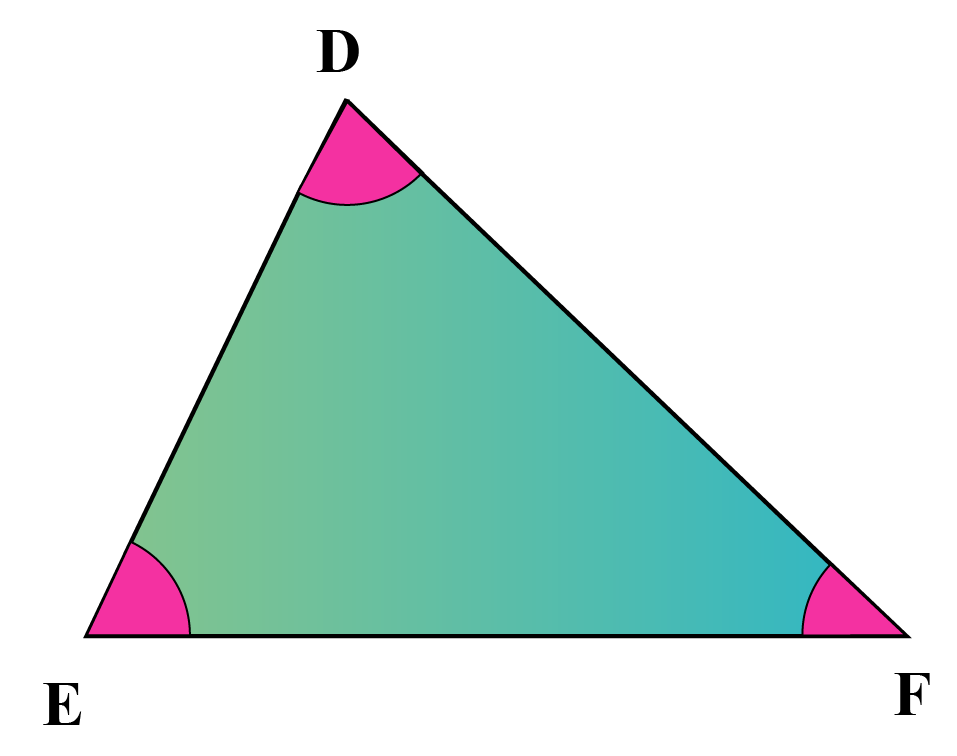it has three vertices: D, E, and F that form the angles of the triangle.

Look at this pentagon below.

Can you count the number of vertices in the given pentagon?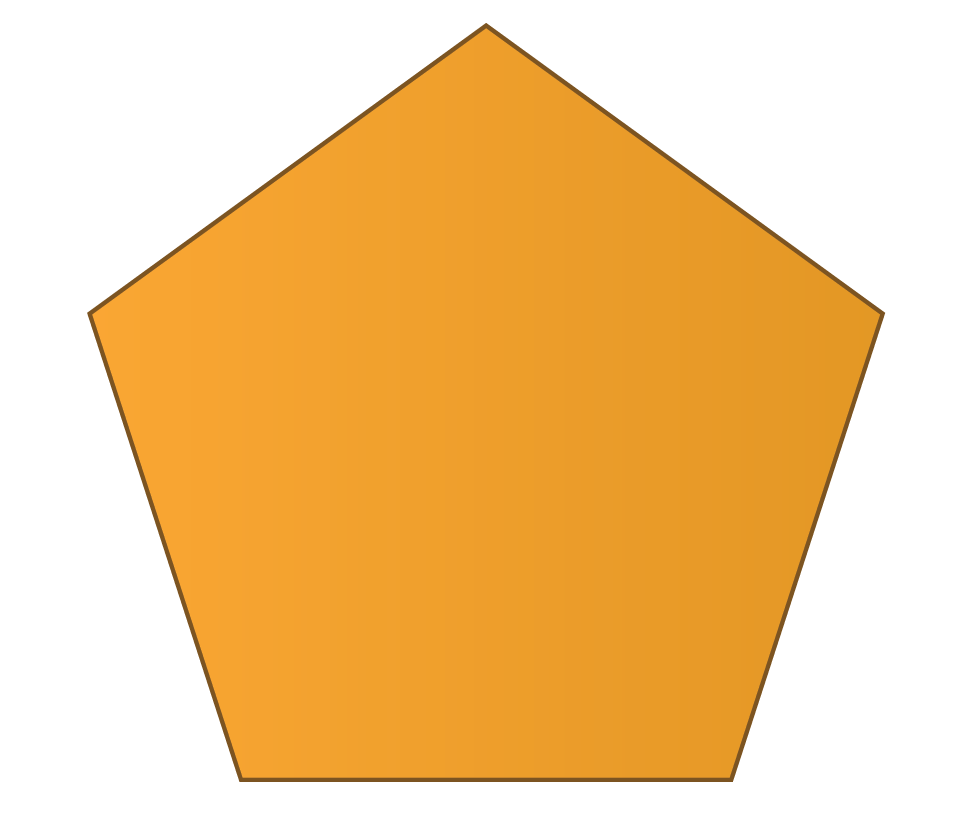## What Is a Vertex In Solid Geometry?

Not just plane shapes, even solid shapes have vertices.

In solid shapes, a vertex is formed where edges meet.

Examples

Look at this cube,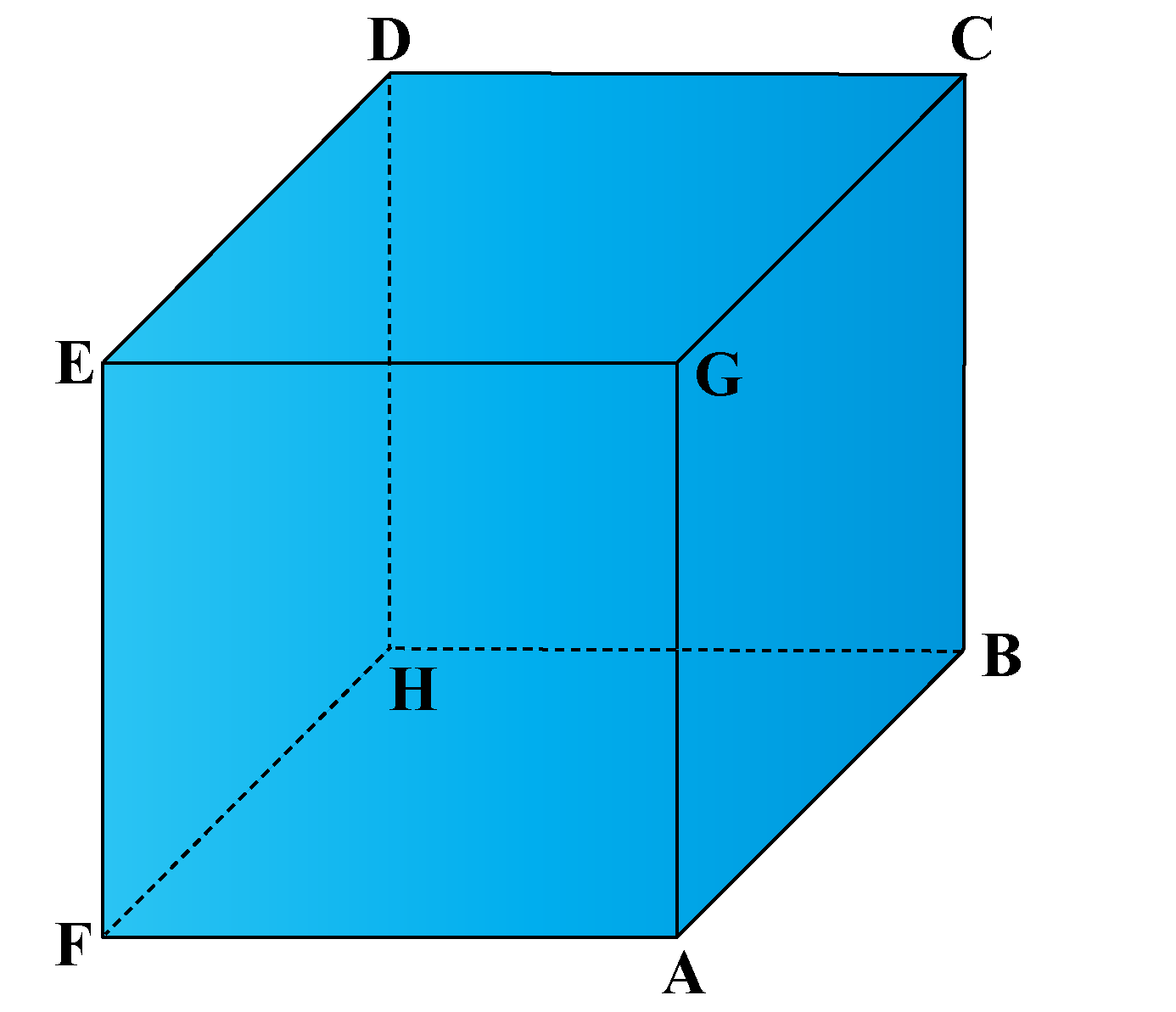A, B, C, D, E, F, G, H are the vertices of the cube.

A tetrahedron has 4 vertices. One is marked. Can you identify the other 3  vertices of the tetrahedron?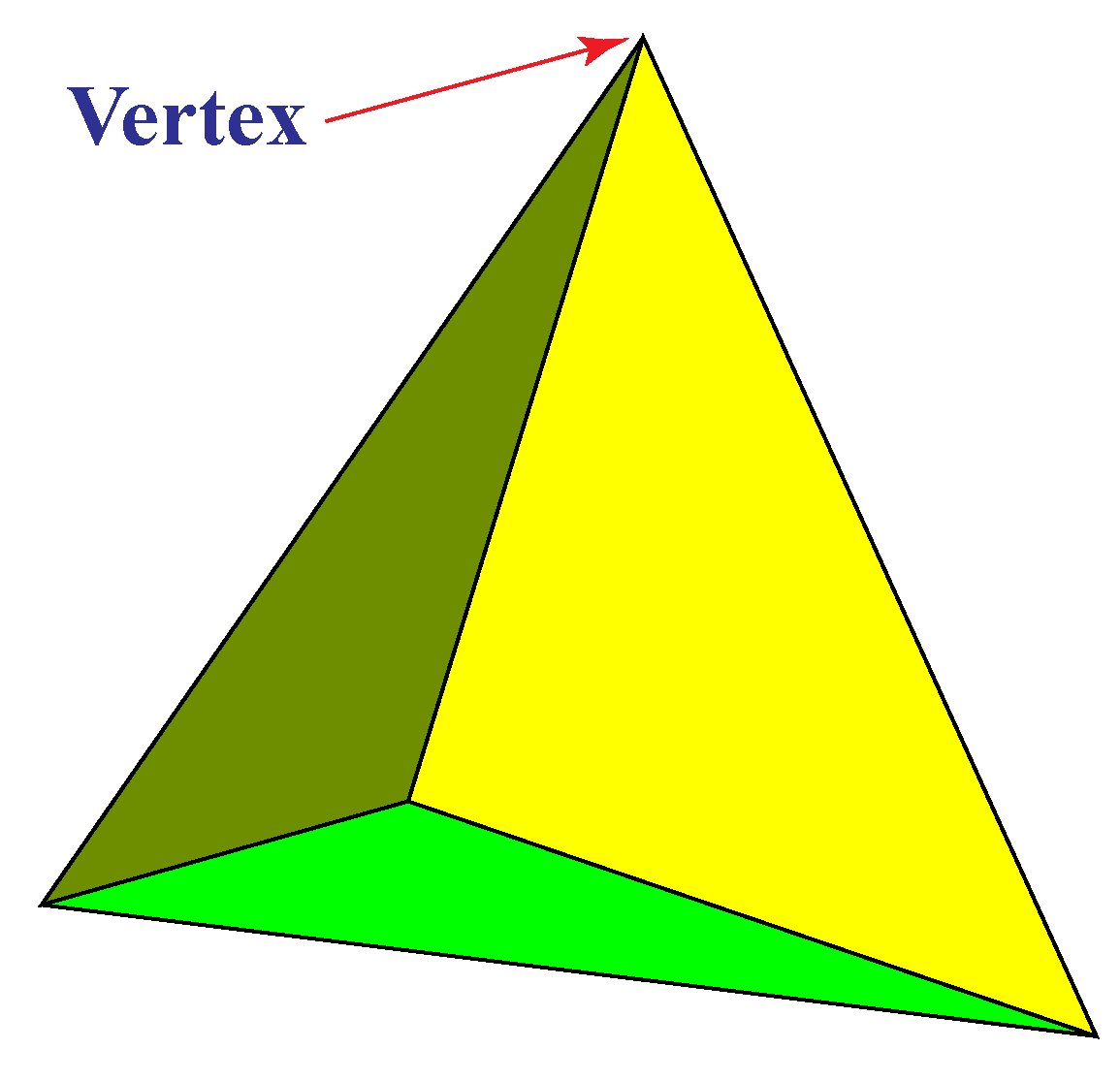We can apply Euler's formula to find the vertices in a solid shape.

Euler's formula is:

 F + V − E = 2

Where,

• F is the number of faces
• V stands for the vertices
• E is the number of edges

## What Is the Vertex of a Parabola?

When we graph a quadratic equation, we get a parabola.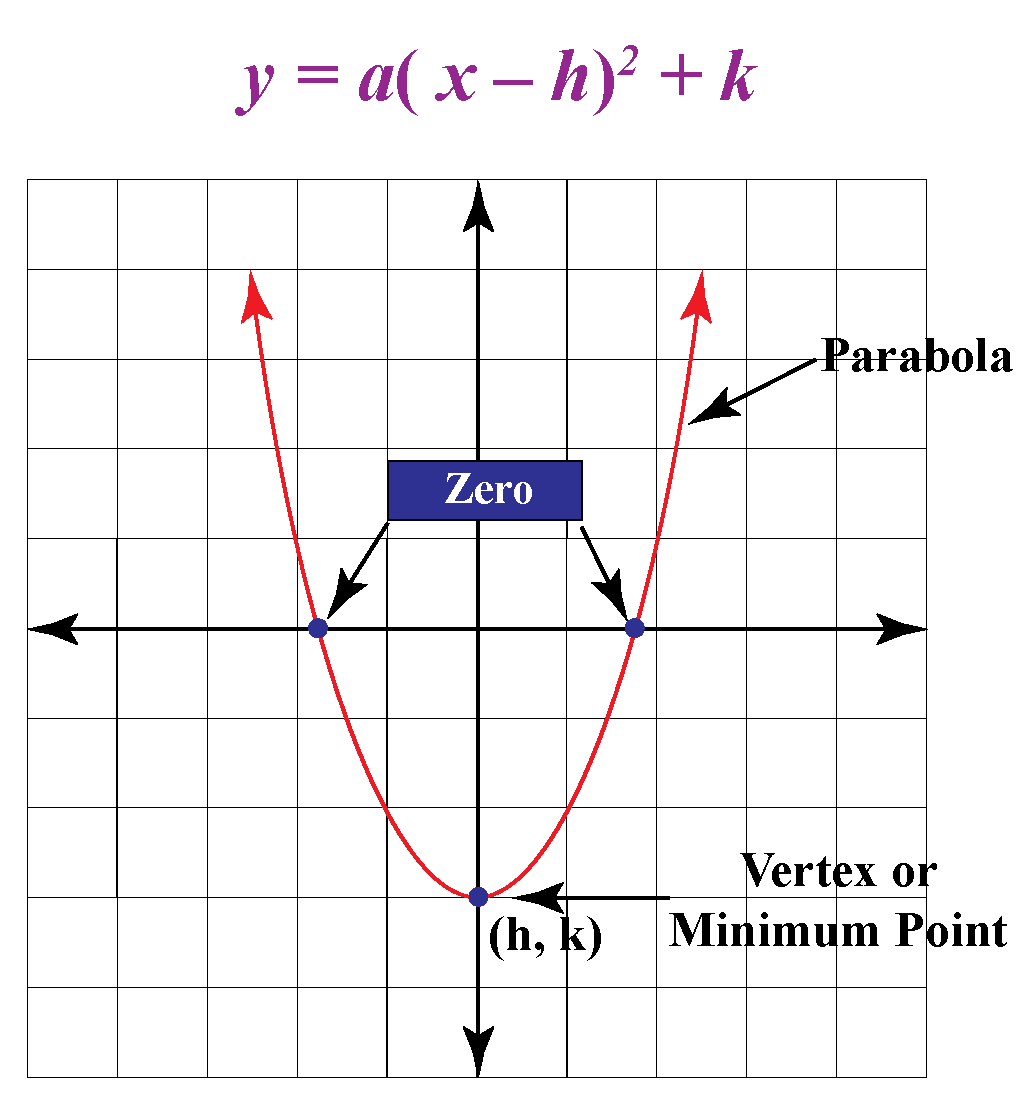The vertex definition of a parabola is the point where exactly it turns.

It is also called the minimum point.

When the parabola , opens down the vertex is  called the maximum point.

The parabola vertex lies at the axis of symmetry.

In the standard form, we write the quadratic equation as $$ax^2 + bx + c$$

In the standard form, the vertex (V) of the parabola is  given by:

 $V \equiv \left( { - \frac{b}{{2a}}, -\frac{D}{{4a}}} \right)$

where D is the discriminant. \begin{align}x = {-b \pm \sqrt{b^2-4ac} \over 2a}\end{align}

The vertex equation of a parabola is of the form $$y = a(x-h)^2 + k$$

The vertex of the parabola  is at the coordinate (h, k)

 Vertex of the parabola (h,k)

Example 1

Graph of $$f\left( x \right) = 1 - 2x -3{x^2}$$ is as shown below . Find the vertex of the parabola.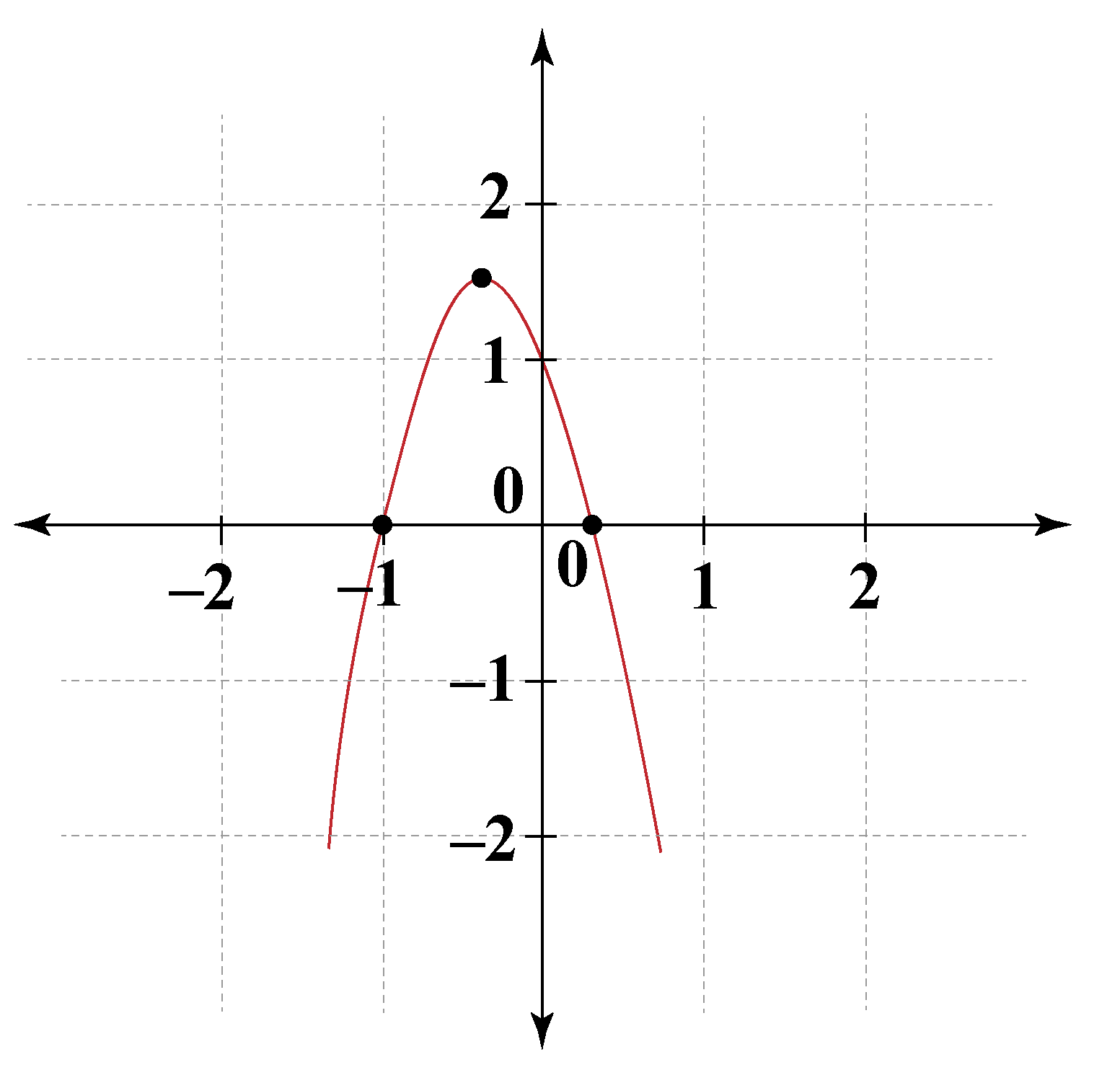Solution

We have:

$\begin{array}{l}a = - 3,\,\,\,b = - 2,\,\,\,c = 1\\ \Rightarrow \,\,\,D = {b^2} - 4ac =16\end{array}$

The coordinates of the vertex are.

$V \equiv \left( { - \frac{b}{{2a}}, -\frac{D}{{4a}}} \right) = \left( { - \frac{1}{3},\frac{4}{3}} \right)$

Note that the parabola will open downward (since $$a$$ is negative), but the vertex has a positive y-coordinate.

 $$\therefore$$ Vertex of the parabola is at $$-\dfrac{1}{3} , \dfrac{4}{3}$$

Example 2

Find the vertex of the parabola  $$y =2(x+3)^2−8$$

Solution

We know that

The vertex equation of a parabola is of the form  $$y = a(x – h)^2 + k$$ where (h,k) is the vertex.

In the given equation, h = 3 and k = - 8

Therefore the vertex of the parabola is (3,-8)

 $$\therefore$$ Vertex V of the parabola is at (3,-8)

## Solved Examples

 Example 1

How many vertices does a rectangle have?

Solution

Vertices are the corners of the rectangle.

A rectangle has 4 corners, and hence it has 4 vertices.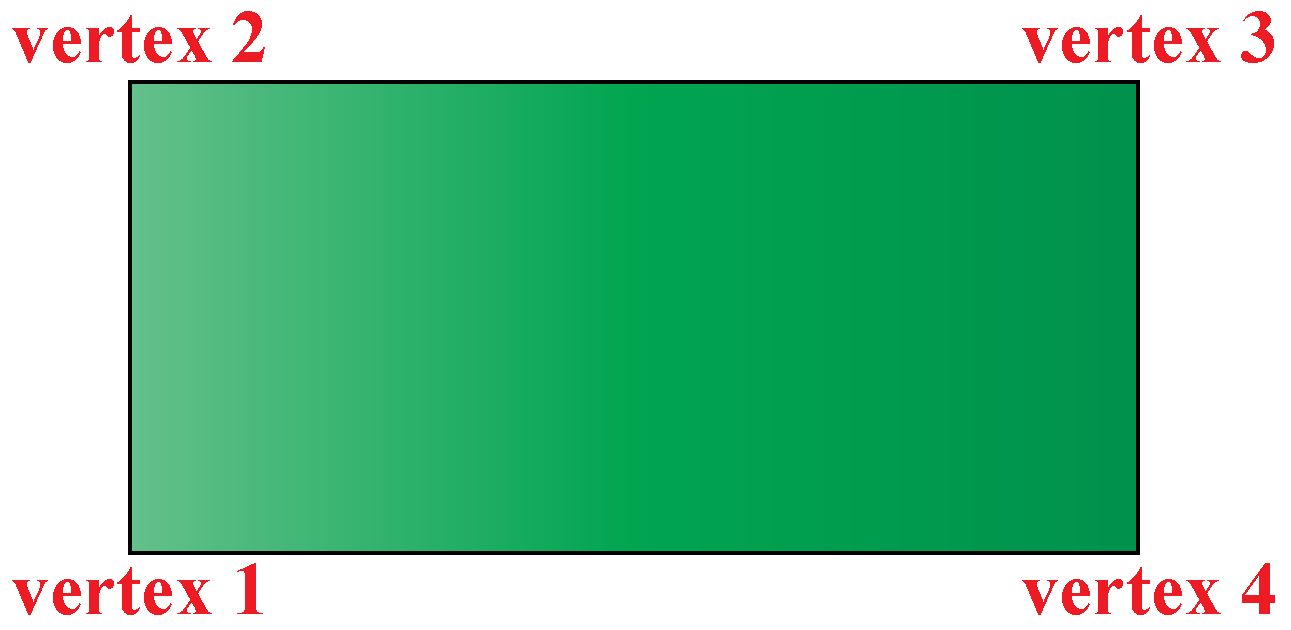$$\therefore$$ A rectangle has 4 vertices.
 Example 2

How many vertices does a circle have?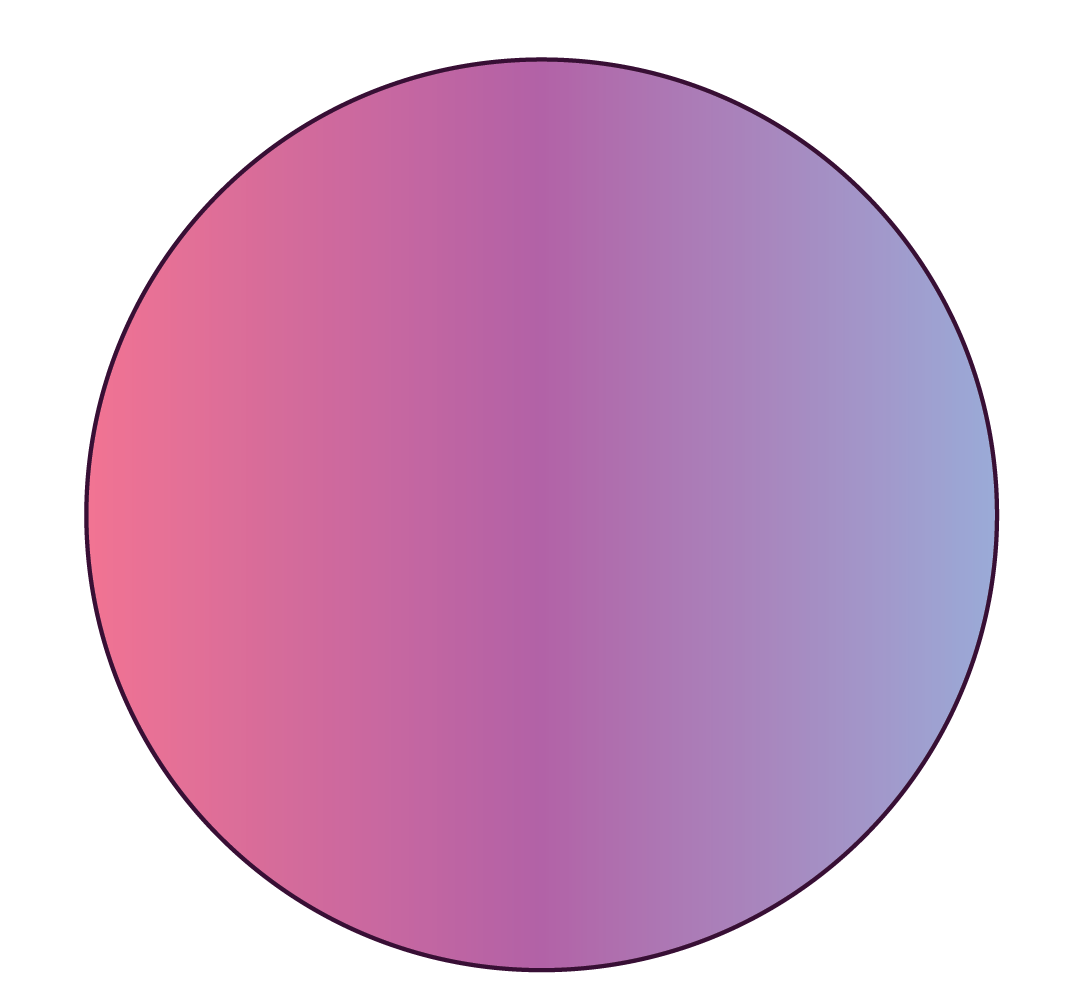Solution

A circle does not have any corner.

Hence, there are no vertices in a circle.

 $$\therefore$$ A circle does not have any vertices.
 Example 3

Tarun said there are as many vertices in a Rubik's cube as in a cuboid.

Is Tarun correct?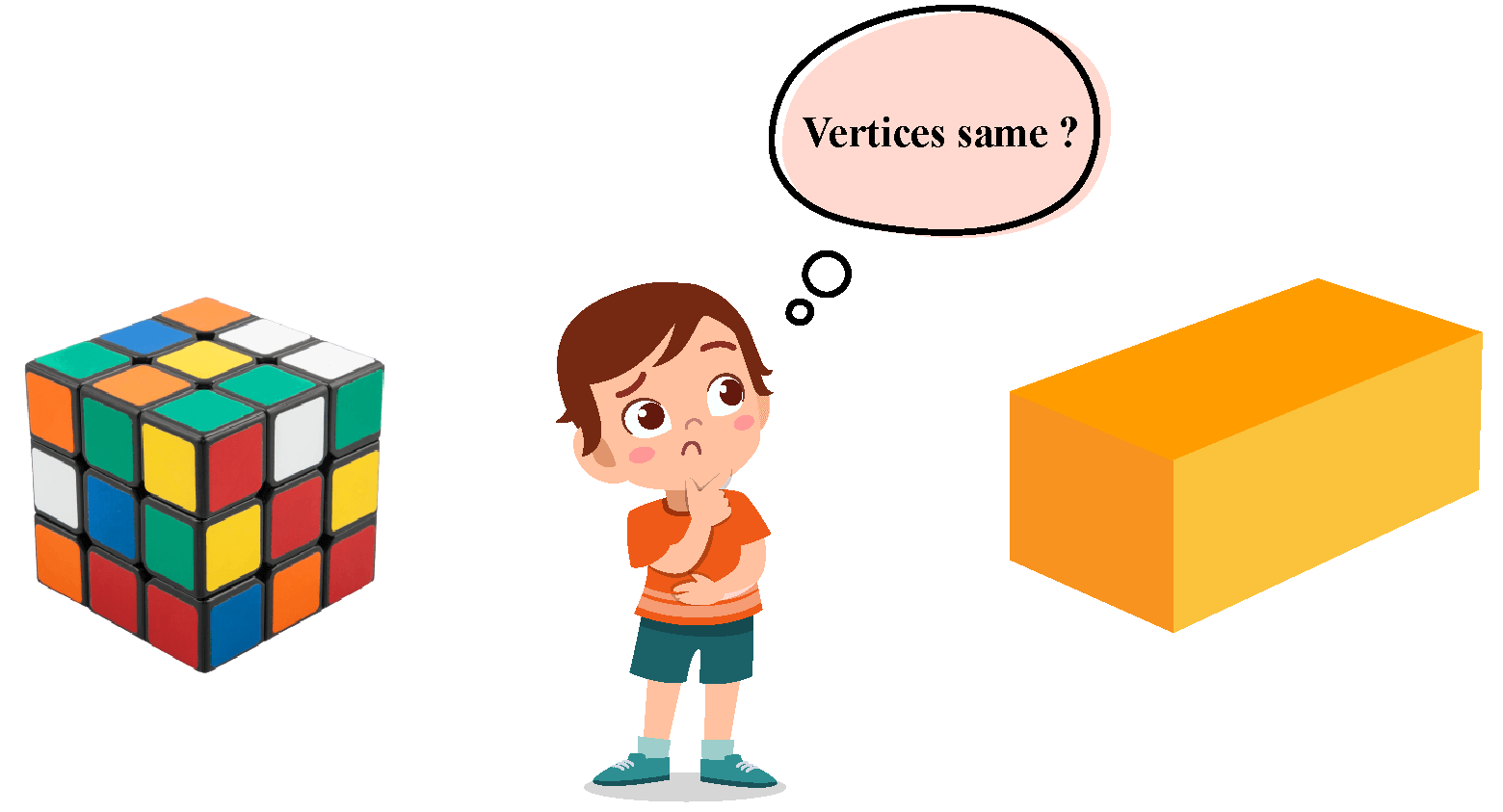Solution

Let us count the number of corners in the Rubik's cube and the cuboid.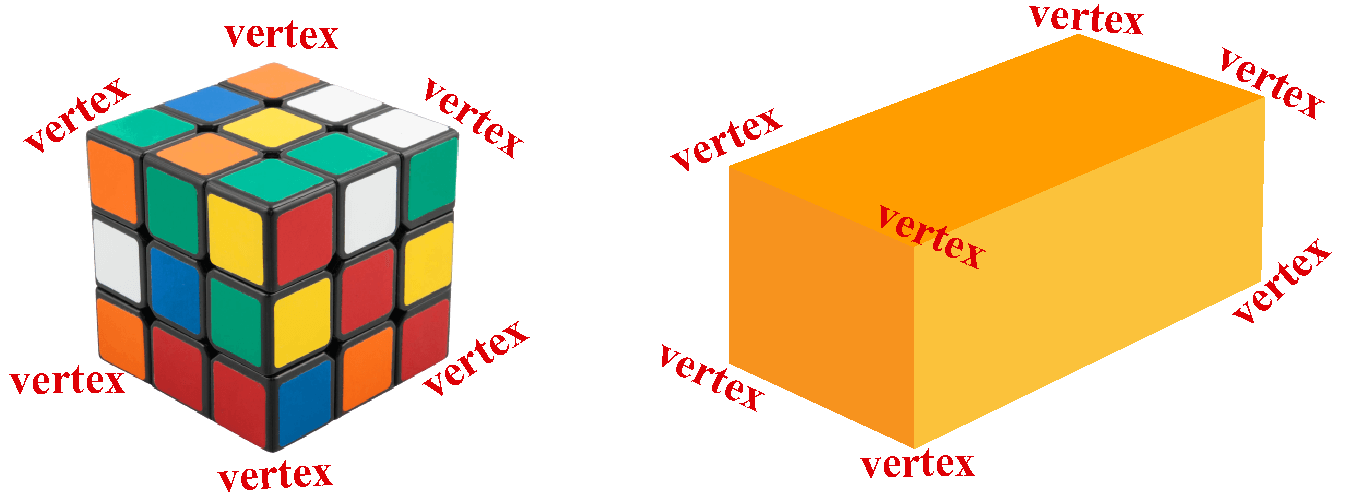Both of them have 8 vertices.

 $$\therefore$$ Tarun is correct. A cuboid and a Rubik's cube have 8 vertices.
 Example 4

Help Manav identify the number of vertices in each of the given shapes.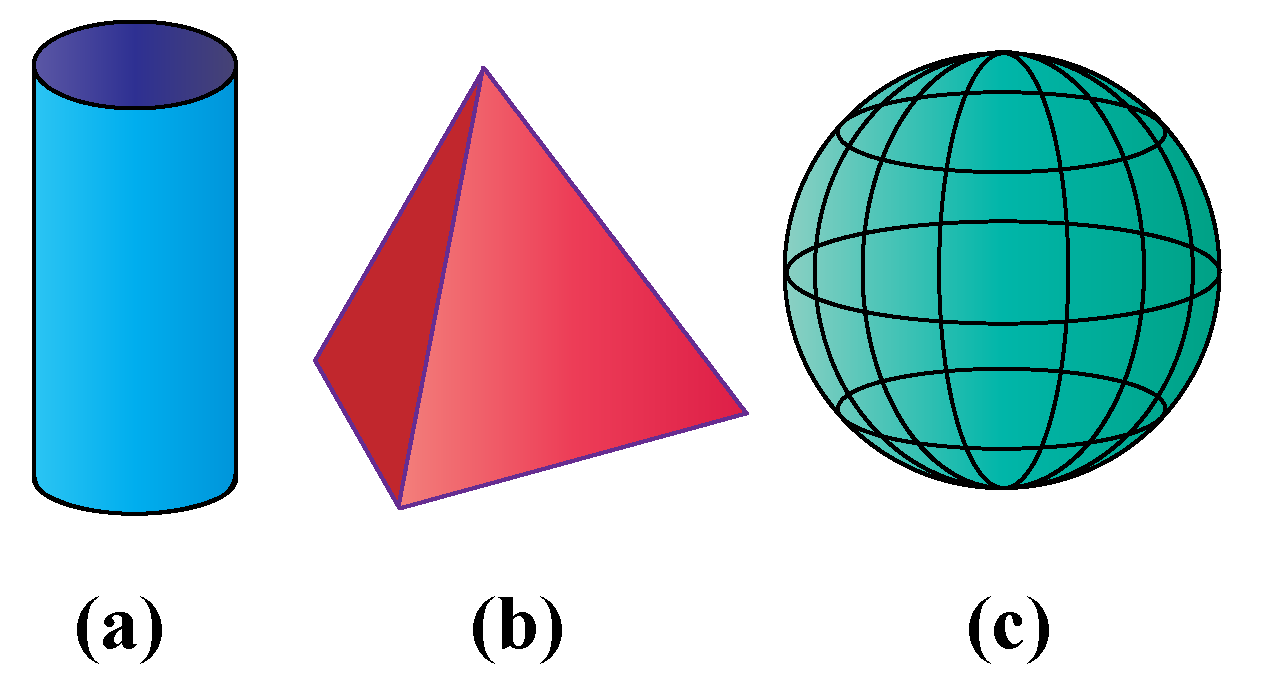Solution

We can count the number of corners in each of the shapes to tell the number of vertices.

Shape Number of Vertices
Cylinder 0
Square pyramid 5
Sphere 0Challenging Questions
1. Does a cone have a vertex?
2. How many vertices will a polygon of n sides have?

## Interactive Questions

Here are a few activities for you to practice. Select/Type your answer and click the "Check Answer" button to see the result.

## Let's Summarize

We hope you enjoyed learning about vertex with the examples and practice questions. Now you will be able to easily solve problems on vertex definition, vertex example, vertex angle, and vertex in a parabola.

At Cuemath, our team of math experts is dedicated to making learning fun for our favorite readers, the students!

Through an interactive and engaging learning-teaching-learning approach, the teachers explore all angles of a topic.

Be it worksheets, online classes, doubt sessions, or any other form of relation, it’s the logical thinking and smart learning approach that we, at Cuemath, believe in.

## 1. What is the meaning of vertex?

Vertex is the point of intersection of edges or line segments.

## 2. Why is the vertex important?

Vertex is important as it defines the high point or low point, as the high point in an isosceles triangle or the minimum point in a parabola.

## 3. What is the vertex of a graph?

Vertex is the node of the graph. It is also the point that defines the node or the maximum or minimum point.

## 4. What is the vertex of an angle example?

The vertex of an angle is the point where two rays begin or meet.Look at the example: Ray1 and Ray 2 originate from vertex O.

More Important Topics
Numbers
Algebra
Geometry
Measurement
Money
Data
Trigonometry
Calculus
More Important Topics
Numbers
Algebra
Geometry
Measurement
Money
Data
Trigonometry
Calculus